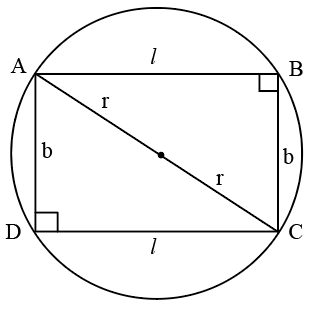Question 13

# All the vertices of a rectangle lie on a circle of radius R. If the perimeter of the rectangle is P, then the area of the rectangle is

Solution$$A=lb$$

$$l^2+b^2=4r^2$$

$$P\ =2\left(l+b\right)$$

$$\frac{P}{2}=l+b$$

Squaring on both the sides, we get

$$\frac{P^2}{4}=l^2+b^2+2lb$$

$$\frac{P^2}{4}=4r^2+2lb$$

$$\frac{P^2}{8}-2r^2=lb$$